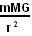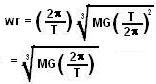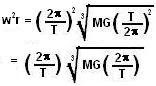## Saturday, October 4, 2008

### Irodov Problem 1.223Let the angular velocity of Earth's rotation about its axis be w. In order for the satellite to be stationary with respect to the Earth's surface (i.e. geostationary), it must be rotating with the same angular velocity w. If the satellite's radius is r, its centripetal acceleration is given bywhere m is the mass of the satellite. The gravitational force acting on the satellite is given by, where M is the Earth's mass. So we have,Here T is the time period of rotation of Earth about its axis (24hrs).
Knowing that,We can calculate this radius r as about 42,225 km. Since the Earth's radius itself is 6378 km, the height of the Geostationary satellite has to be about 35,900 km above the Earth's surface.

The velocity of the satellite is given by wr and its acceleration is its centripetal acceleration and are given by,The numerical value can be obtained as 3.071 Km/s.

The centripetal acceleration is given by,The numerical value is given by 0.223 m/s^2.